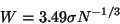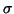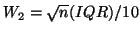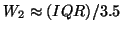Next: Sampling and Sub-Sampling Up: Implementation Previous: Parameterisation

## Histogram Bin Size

Another important choice to make for most cost functions is the histogram bin size, or equivalently, number of bins. All the cost functions investigated in section 3.2 require some form of intensity binning. For the Correlation Ratio and Woods function the intensity bins are only used for the reference volume in order to determine the iso-sets, whereas for the entropy-based functions, intensity binning occurs for both images.

The number of intensity bins determines two things: (1) how good the statistics will be in reflecting the ideal, continuous distribution and (2) the effective fidelity of the intensities (that is, using 256 bins is equivalent to that image having an 8 bit intensity range). In most cases it is the first point that is of most interest, as the Signal to Noise Ratio in a typical image limits the maximum practical fidelity.

Determining how many histogram bins should be used for estimating distributions is a problem in non-parametric statistics, although histogram-based methods are not the only form of distribution estimation (for an overview see [Izenman, 1991]). However, histogram-based methods are the most practical as other methods usually involve too much computational overhead to be useful for this problem.

It has been shown [Scott, 1979] that the optimal histogram bin size, which provides the most efficient, unbiased estimation of the probability density function, is achieved when:(38)

where W is the width of the histogram bin,is the standard deviation of the distribution and N is the number of available samples. In practice, the estimated standard deviation, s, must be used. A similar, but more robust, result was also obtained by Freedman and Diaconis (summarised in [Izenman, 1991]), which gives the bin width as:

 W = 2 (IQR) N-1/3 (39)

where IQR is the interquartile range (the 75th percentile minus the 25th percentile).

In both formulations the width is proportional to N-1/3. However, it is common practice for the number of histogram bins (and hence the size) to be set as a pre-defined constant in a registration method. This results in the statistics becoming less precise as the sub-sampling is increased. For example, with 8mm cubed voxels and a 200mm cubed FOV, there are 15625 voxels in the image, and if the expected number of samples per bin in a joint histogram is to be at least 10, then there must be 1562 bins or less, or less than 40 intensity bins per image. However, it is not unusual to find methods that use 256 bins for this data, which results in an average of 0.2 samples per bin which will result in very poor statistics, and therefore less smooth cost functions.

For the method proposed here, the histogram bin size is set proportional to N-1/3. This means that at a resolution with voxel size of n mm cubed, the number of voxels, or samples, is N = (200/n)3. Therefore, the histogram bin size is given by W = n (IQR)/100. However, this is only true for 1D histograms, where intensity bins are only used for a single image, such as for Correlation Ratio and the Woods function. For the entropy-based functions, a 2D joint histogram is required and so the result must be generalised.

The two easiest ways of generalising the above result are (1) to keep the bin width the same or (2) to keep the average number of samples per bin the same. Given that the number of bins within the IQR is now (IQR)2/W2 and the estimated number of samples per bin is N W2 / (2 IQR3), the two generalisations give bin widths of W1 = n (IQR)/100 andrespectively. However, this gives a very large bin width for the latter case, so that for the 8mm resolution, W1 = (IQR)/12.5 as compared to, whereas the expected number of samples per bin are 50 and 625 respectively. Therefore, the former generalisation ensures a sufficient number of samples per bin whilst providing a better fidelity, and so this is the one that is chosen.

In practice, the number of intensity bins used per image at resolution n is 256/n. This is equivalent to estimating the IQR to 39% of the total intensity range. In addition, this gives the same number of bins at a 1mm resolution (that is, 256) as is common in many other methods, while maintaining near optimal sampling requirements and a reasonable level of fidelity, in keeping with the expected Signal to Noise Ratio.Next: Sampling and Sub-Sampling Up: Implementation Previous: Parameterisation
Mark Jenkinson
2000-05-10